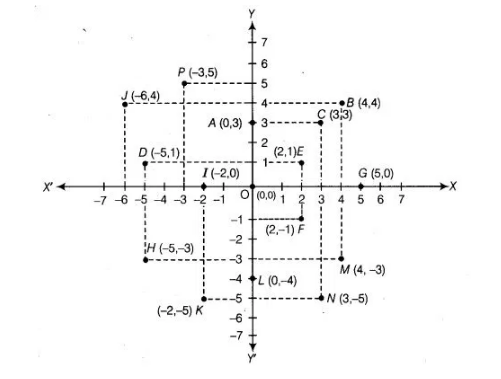# From the given figure, answer the following questions`
Question:

From the given figure, answer the following questions(i) Write the points whose abscissa is 0.

(ii) Write the points whose ordinate is 0.

(iii) Write the points whose abscissa is – 5,

Solution:

(i) We know that, the point whose abscissa is 0 will lie on Y-axis. So, the required points whose abscissa is 0 are A, L and O.

(ii) We know that, the point whose ordinate is 0 will lie on X-axis. So, the required points, whose ordinate is 0 are G,l and O.

(iii) Here, abscissa ‘-5’ is negative, which shows that point with abscissa -5 will lie in II and III quadrants. So, the required points whose abscissa

is -5, are D and H.

Note: We know that, origin O is the intersection point of both axes. So, we can consider it on X-axis as well as on Y-axis.